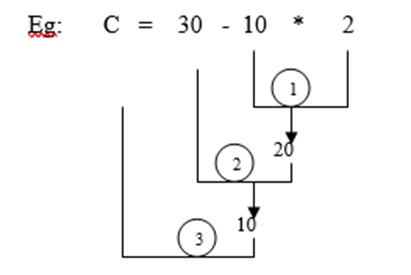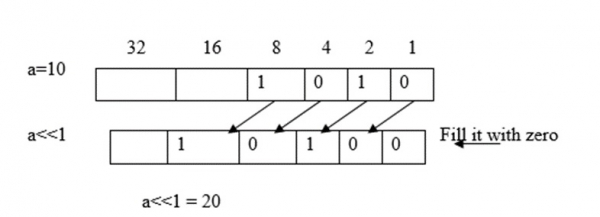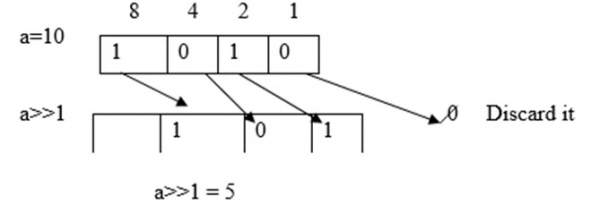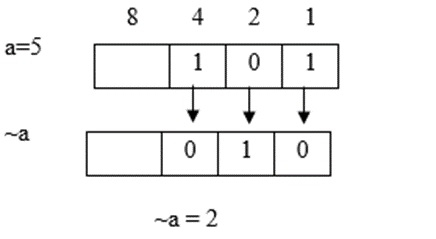# What are different operators and expressions used in C language?

Operator performs an operation on data. They are classified into following −

• Arithmetic operators.
• Relational operators.
• Logical operators.
• Assignment operators.
• Increment and decrement operators.
• Bitwise operators.
• Conditional operators.
• Special operators.

## Arithmetic operator

These operators are used for numerical calculations (or) to perform arithmetic operations like addition, subtraction etc.

OperatorDescriptionExamplea=20,b=10Output
-subtractiona-b20-1010
*multiplicationa*b20*10200
/Divisiona/b20/102(quotient)
%Modular Divisiona%b20%100 (remainder)

## Program

Following is the C program for arithmetic operator −

#include<stdio.h>
main ( ){
int a= 20, b = 10;
printf (" %d", a+b);
printf (" %d", a-b);
printf (" %d", a*b);
printf (" %d", a/b);
printf (" %d", a%b);
}

## Output

When the above program is executed, it produces the following result −

30
10
200
20

## Relational operators

These are used for comparing two expressions.

OperatorDescriptionExamplea=20,b=10Output
<less thana<b10<201
<=less than (or) equal toa<=b10<=201
>greater thana>b10>200
>=greater than (or) equal toa>=b10>=200
==equal toa==b10==200
!=not equal toa!=b10!=201

The output of a relational expression is either true (1) (or) false (0).

## Program

Following is the C program for relational operator −

#include<stdio.h>
main ( ){
int a= 10, b = 20;
printf (" %d", a<b);
printf (" %d", a<=b);
printf (" %d", a>b);
printf (" %d", a>=b);
printf (" %d", a = =b);
printf (" %d", a ! =b);
}

## Output

When the above program is executed, it produces the following result −

1 1 0 0 0 1 1 1

## Logical Operators

These are used to combine 2 (or) more expressions logically.

They are logical AND (&&) logical OR ( || ) and logical NOT (!)

exp1exp2exp1&&exp2
TTT
TFF
FTF
FFF

Logical AND(&&)

exp1exp2exp1||exp2
TTT
TFT
FTT
FFF

Logical OR(||)

exp!exp
TF
FT

Logical NOT(!)

OperatorDescriptionExamplea=20,b=10Output
&&logical AND(a>b)&&(a<c)(10>20)&&(10<30)0
||logical OR(a>b)||(a<=c)(10>20)||(10<30)1
!logical NOT!(a>b)!(10>20)1

## Program

Following is the C program for logical operator −

#include<stdio.h>
main ( ){
int a= 10, b = 20, c= 30;
printf (" %d", (a>b) && (a<c));
printf (" %d", (a>b) | | (a<c));
printf (" %d", ! (a>b));
}

## Output

When the above program is executed, it produces the following result −

0 1 1

## Assignment operators

It assigns a value to a variable. The types of assignment operators are −

• Simple assignment.
• Simple assignment.
OperatorDescriptionExample
=simple assignmenta=10
+=,-=,*=,/=,%=compound assignmenta+=10"a=a+10
a=10"a=a-10

## Program

Following is the C program for assignment operator −

#include<stdio.h>
main ( ){
int a= 10,;
printf (" %d", a);
printf (" %d", a+ =10);
}

## Output

When the above program is executed, it produces the following result −

10
20

## Increment and decrement operator

Let us understand what is an increment operator.

### Increment operator (++)

This operator increments the value of a variable by 1

The two types include −

• pre increment
• post increment

If we place the increment operator before the operand, then it is pre-increment. Later on, the value is first incremented and next operation is performed on it.

For example,

z = ++a; // a= a+1
z=a

If we place the increment operator after the operand, then it is post increment and the value is incremented after the operation is performed.

For example,

z = a++; // z=a
a= a+1

## Example

Following is the C program for increment operator −

ProgramProgram
main() {
int a= 10, z;
z= ++a;
printf("z=%d", z);
printf("a=%d", a);
}
main() {
int a= 10, z;
z= a++;printf("z=%d", z);
printf("a=%d", a);
}
OutputOutput
z= 11
a=11
z= 10
a=11

## Decrement operator − (- -)

It is used to decrement the values of a variable by 1.

The two types are −

• pre decrement
• post decrement

If the decrement operator is placed before the operand, then it is called pre decrement. Here, the value is first decremented and then, operation is performed on it.

For example,

z = - - a; // a= a-1
z=a

If the decrement operator is placed after the operand, then it is called post decrement. Here, the value is decremented after the operation is performed.

For example,

z = a--; // z=a
a= a-1

 main() {    int a= 10, z;    z= --a;    printf("z=%d", z);    printf("a=%d", a); } main() {    int a= 10, z;    z= a--;    printf("z=%d", z);    printf("a=%d", a); } Output Output z= 9 a=9 z= 10 a=9

## Bitwise Operator

Bitwise operators operate on bits.

OperatorDescription
&Bitwise AND
|Bitwise OR
^Bitwise XOR
<<Left Shift
>>Right shift
~One's Complement

Bitwise AND
aba&b
000
010
100
111

Bitwise OR
aba|b
000
011
101
111

Bitwise XOR
aba^b
000
011
101
110## Program

Following is the C program for bitwise operator −

#include<stdio.h>
main ( ){
int a= 12, b = 10;
printf (" %d", a&b);
printf (" %d", a| b);
printf (" %d", a ^ b);
}

## Output

When the above program is executed, it produces the following result −

8 14 6

## Left Shift

If the value is left shifted one time, then its value gets doubled.

For example, a = 10, then a<<1 = 20## Right shift

If the value of a variable is right shifted one time, then its value becomes half the original value.

For example, a = 10, then a>>1 = 5## Ones complement

It converts all ones to zeros and zeros to ones.

For example, a = 5, then ~a=2 [only if 4 bits are considered].## Program

Following is another C program for bitwise operator −

#include<stdio.h>
main ( ){
int a= 20, b = 10,c=10;
printf (" %d", a<<1);
printf (" %d", b>>1);
printf (" %d", ~c);
}

## Output

When the above program is executed, it produces the following result −

40
5
11

### Signed

1’s complement = - [ give no +1]

For example, ~10 = - [10+1] = -11

~-10 = - [-10+1] = 9

### Unsigned

1’s complement = [65535 – given no]

## Conditional operator (? :)

It is also called ternary operator.

The syntax is as follows −

exp1? exp2: exp3

If exp1 is true, exp2 is evaluated. Otherwise, exp3 is evaluated. Or in the form of if-else.

if (exp1)
exp2;
else
exp3;

## Program

Following is the C program for conditional operator −

#include<stdio.h>
main ( ){
int z;
z = (5>3) ? 1:0;
printf ("%d",z);
}

## Output

When the above program is executed, it produces the following result −

## Special operations

Some of the special operations are comma, ampersand (&), size of operators.

• Comma ( , ) − It is used as separator for variables. For example; a=10, b=20
• Size of ( ) − It used to get the size of a data type of a variable in bytes.

## Program

Following is the C program for special operations −

#include<stdio.h>
main ( ){
int a=10;
float b=20 ;
printf (" a= %d b=%f", a,b );
printf (" a address =%u " , &a ) ;
printf (" b address =%u" ,&b ) ;
printf ("a size = %ld" , sizeof (a) ) ;
printf ( "b size = %ld ", sizeof (b) ) ;
}

## Output

When the above program is executed, it produces the following result −

a=10 b=20.00
Address of a =1 2 3 4
Address of b = 5 6 7 8 Only for this example
Size of a = 4 bytes
Size of b = 4 bytes

Updated on: 11-Mar-2021

8K+ Views